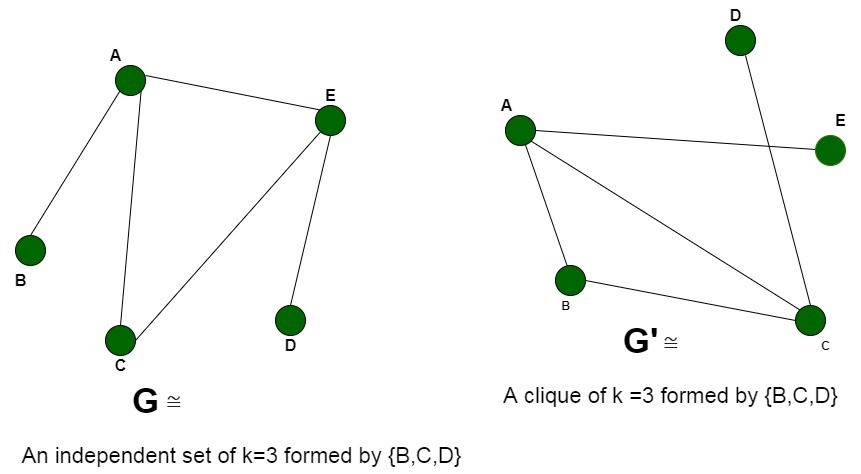Open in App
Not now

# Proof that Clique Decision problem is NP-Complete | Set 2

• Last Updated : 01 Jul, 2020

Prerequisite: NP-Completeness, Clique problem.

A clique in a graph is a set of vertices where each vertex shares an edge with every other vertex. Thus, a clique in a graph is a subgraph which is a complete graph.
Problem: Given a graph G(V, E) and an integer K, the problem is to determine if the graph contains a clique of size K.

Explanation:
An instance of the problem is an input specified to the problem. An instance of the Clique problem is a graph G (V, E) and a positive integer K, and the problem is to check whether a clique of size K exists in G. Since an NP-Complete problem, by definition, is a problem which is both in NP and NP-hard, the proof for the statement that a problem is NP-Complete consists of two parts:

1. The problem itself is in NP class
2. All other problems in NP class can be polynomial-time reducible to that.
(B is polynomial-time reducible to C is denoted as)

If the 2nd condition is only satisfied then the problem is called NP-Hard.

But it is not possible to reduce every NP problem into another NP problem to show its NP-Completeness all the time. That is why if we want to show a problem is NP-Complete we just show that the problem is in NP and any NP-Complete problem is reducible to that, then we are done, i.e. if B is NP-Complete andfor C in NP, then C is NP-Complete.

In this article, we will prove that the Clique Detection Problem is NP-Complete by the help of Independent Set problem, which is NP-Complete. Refer to Proof that Clique Decision problem is NP-Complete, for the proof with the help of Boolean Satisfiability Problem.

1. Clique Problem is in NP
If any problem is in NP, then, given a ‘certificate’, which is a solution to the problem and an instance of the problem (a graph G and a positive integer K, in this case), we will be able to verify (check whether the solution given is correct or not) the certificate in polynomial time.

The certificate is a subset V’ of the vertices, which comprises the vertices belonging to the clique. We can validate this solution by checking that each pair of vertices belonging to the solution are adjacent, by simply verifying that they share an edge with each other. This can be done in polynomial time, that is O(V +E) using the following strategy for graph G(V, E):

```flag=true
For every pair {u, v} in the subset V’:
Check that these two
vertices {u, v} share an edge
If there is no edge,
set flag to false and break
If flag is true:
Solution is correct
Else:
Solution is incorrect
```
2. Clique Problem is NP-Hard
To prove that the clique problem is NP-Hard, we take the help of a problem that is already NP-Hard and show that this problem can be reduced to the Clique problem.
For this, we consider the Independent Set problem, which is NP-Complete (and hence NP-Hard). Every instance of the independent set problem consisting of the graph G (V, E) and an integer K can be converted to the required graph G’ (V’, E’) and K’ of the Clique problem. We will construct the graph G’ by the following modifications:
V’ =V, that is all the vertices of graph G are a part of the graph G’
E’ = complement of the edges E, that is, the edges not present in the original graph G.
The graph G’ is the complementary graph of G. The time required to compute the complementary graph G’ requires a traversal over all the vertices and edges.
Time complexity: O (V+E)

We will now prove that the problem of computing the clique indeed boils down to the computation of the independent set. The reduction can be proved by the following two propositions:

• Let us assume that the graph G contains a clique of size K. The presence of clique implies that there are K vertices in G, where each of the vertices is connected by an edge with the remaining vertices. This further shows that since these edges are contained in G, therefore they can’t be present in G’. As a result, these K vertices are not adjacent to each other in G’ and hence form an Independent Set of size K.
• We assume that the complementary graph G’ has an independent set of vertices of size K’. None of these vertices shares an edge with any other vertices. When we complement the graph to obtain G, these K vertices will share an edge and hence, become adjacent to each other. Therefore, the graph G will have a clique of size K.Thus, we can say that there is a clique of size K in graph G if there is an independent set of size K in G’ (complement graph). Therefore, any instance of the clique problem can be reduced to an instance of the Independent Set problem. Thus, the clique problem is NP-Hard.

Conclusion:

Hence, the Clique Decision problem is NP-Complete

My Personal Notes arrow_drop_up
Related Articles